Vasile BRĂTIAN

# Evaluation of Options using the Monte Carlo Method and the Entropy of Information

In the present paper, there are presented, theoretical and applicative, two issues: the evaluation of the European options using the Monte Carlo method and the measurement of the entropy of information for the price of the underlying asset of the option. The underlying asset used in our analyses is the share of Compa SA. Through Monte Carlo simulations, scenarios are created on the random evolution of the underlying asset, and the valuation of the option on the underlying asset is made using the Feynman-Kač theorem. The distribution we use is lognormal. Also, in the paper is measured the entropy of information of Shannon type. The measurement of the entropy of information of the stock market price of the underlying asset is calculated annually, considering the stock market price in this case as a discreet random variable.

### 1. Introduction

One of the most used methods for evaluating a derivative of the nature of the option is the Monte Carlo simulation. The use of the Monte Carlo simulation to create scenarios on the evolution of the underlying asset price and the Feynman-Kac theorem can be a very useful way for practitioners to determine solutions for Call and Put.So, one of the problems of this paper is to describe theoretically and practically how this can be achieved. The solution for the assessment of options was given by Fischer Black and Myron Scholes (1973) through an equation with second order partial derivatives, parabolic, and represents a major scientific contribution to finance, creating an industry of one million billion dollars.

Mainly, solving the Black-Scholes equation can be accomplished by two methods (Negrea and Damian, 2015, p. 333): either by using the classical theory of solving Partial Derivatives Equations of parabolic type, or by using the Feynman-Kac representation theorem.

Regarding the Feynman-Kac theorem, we can say that it is extremely useful in solving the Black-Scholes equation, representing a method of solving the partial derivatives equations that are very convenient from a technical point of view. In fact, the theorem provides the probabilistic solution of the Black-Scholes partial derivative equation, and the explicit calculation of expectation of the Feynman-Kac theorem offers the possibility of determining the analytical solutions for Call and Put in the Black-Scholes model.

Also, along with the above issues, the paper describes how to determine the entropy of information on the price of the underlying asset. In this respect, our objective here is to observe the evolution of the entropy of information of the price of the underlying asset from one year to the next and the way in which we will determine the annual entropy of information is based on the information theory methodology proposed by Claude E. Shannon (1948).

The use of the concept of entropy of information to designate the amount expressed by the appearance of a symbol in the set of possible symbols was suggested to Shannon by John von Neumann. Shannon's theory can be formulated as follows: every symbol we use in communication is a macro state to which a number of different micro states can correspond, meaning the other symbols in the set of possible symbols. So, the occurrence of a symbol thus contains a surprise value that derives from the fact that it excluded the occurrence of one of the other symbols of the set.

In the above sense, this theory makes it possible the precise formulation of the difficulty of communication: a symbol is a macro state whose surprise value is determined by how many micro states (i.e., individual symbols) correspond to this macro state (Nørretranders, 2009, p. 48).

This being said, we are also making a last statement regarding the case study from the paper, namely that the underlying asset to which we refer is of the nature of the stock listed on the Bucharest Stock Exchange, namely shares of Compa SA (Bvb.ro, 2018a), which does not distribute dividends.

### 2. Literature Review

A synthesis of the works using the Monte Carlo method in the evaluation of the options is found in our previously published article, which refers to the evaluation of the various types of options (Bratian, 2017): for options of European type, Boyle (1977); for options of Asian type, Broadie and Glasserman (1996); for options of American type, Langstaff and Schwartz (2001); we also mention here Charnes (2000), Bolia and Juneja (2005), Giles (2007), Bingqian Lu (2011), Jespersen (2015).

Measuring entropy on capital markets is the subject of many papers, being a matter of scientific relevance. Here are some of these works that deal with this issue: Nawrocki and Harding (1986); Cabrales et al. (2011); Bentes and Menezes (2012); Maasoumi and Racine (2002); Zhou et al. (2013); Sheraz et al. (2015); Pele et al. (2017). A synthesis work on the development of information theory over a period of fifty years is that of Verdú (1998).

### 3. Methodology

#### 3.1. Evaluating Options using the Feynman-Kac Theorem

In the model proposed by Black and Scholes for evaluating options, we have a number of assumptions, namely (Black and Scholes, 1973, p. 740):

“the short-term interest rate is known and is constant through time; the stock price follows a random walk in continuous time with a variance rate proportional to the square of the stock price. Thus, the distribution of possible stock prices at the end of any finite interval is lognormal. The variance rate of the return on the stock is constant; the stock pays no dividends or other distributions; the option is "European," that is, it can only be exercised at maturity; there are no transaction costs in buying or selling the stock or the option; it is possible to borrow any fraction of the price of a security to buy it or to hold it, at the short-term interest rate; there are no penalties to short selling. A seller who does not own a security will simply accept the price of the security from a buyer, and will agree to settle with the buyer on some future date by paying him an amount equal to the price of the security on that date”.

From the above hypotheses, the price of the underlying asset follows a random evolution given by the following equation:(1)

where:

S = price of the underlying asset;

µ = drift;

σ = volatility of the underlying asset;

dB = Brownian motion;

t = time.

The value of the underlying asset in the Black-Scholes methodology follows a random neutral risk evolution. This the dynamics can be determined by Girsanov's theorem (see Bratian, 2017). Under these conditions, the dynamic equation is:(2)

where: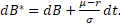In extenso, to make it an Itô process, () to be martingale, its drift must be null. Thus, in order to use the martingale property, it can be made a probability change that transforms this process into a stochastic integral. The change can be achieved through Girsanov's theorem.

The theorem tells us that (Bratian, 2017): either a Brownian movementdefined on the probability field (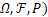,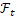a filter generated by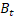and the stochastic process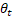. We define the probability measure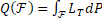, wherewith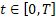. Then the process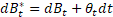is a Brownian motion relative to the measure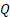. Hence, in a neutral risk-based universe, the expected return on the underlying asset is the risk-free interest rate (). Change of probability (of the realin the risk-neutral) recovers the Brownian motion, changes the drift, but does not change the diffusion coefficient. Volatility is the same on both real and risk-free markets.

We also know from the assumptions that the distribution of the underlying asset is lognormal. Therefore, the neutral risk stochastic differential equation above can be written as follows:

Let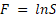. Differential of function, developing in Taylor series, is:=

==

=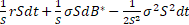=

==

=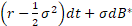=

=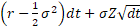(3)

where: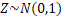Note: In the above development we used heuristics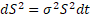.

Expression (3) can be integrated and we will have: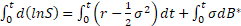=

=(4)

As a result, the solution for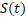is: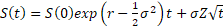, (5)

and over a step of time (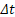) we will have: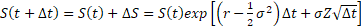(6)

Note: Equation (6) will be used by us to create scenarios on the evolution of the underlying asset.

Next, the Black-Scholes equation tells us that: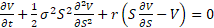(7)

We know that the value of the option is a function of the underlying asset at the time of maturity (T). As a result, it must be written a function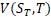representing the profit/loss on maturity for Call and Put.

For the Call option we have (Wilmott, 2007, pp. 175-179):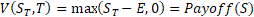, (8)

and for Put we have: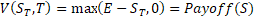(9)

where: E = strike price; T = the time of maturity.

The only probabilistic solution of equation (7) is given using the Feynman – Kac theorem. The Feynman-Kac theorem tells us that if S is a variable that follows a stochastic process, defined as in relation (2), and either a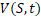function, differentiable from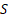andthus:, then putting the condition to the limitandconstant, we will have as the unique solution of the partial-derivative equation the following formal expression:(10)

As a result, from the equations (8), (9) and (10) we can write (Bratian, 2017):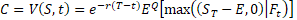, for Call (11), for Put (12)

In an exhaustive way, the Feynman-Kac theorem tells us that in a neutral risk universe, equation (7) can be written as such (Negrea, 2006, pp. 136-137):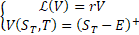(12)

where,is the Dynkin operator associated with the diffusion process given by the following stochastic differential equation: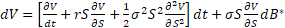(13)

where: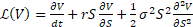.

As a result, the stochastic differential equation that checks the price of the option can be written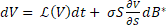and knowing that, then: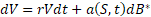(14)

where: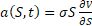.

Equation (14) has as unique solution the following formal expression:(15)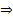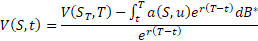(16)

Then, applying, under a neutral risk probability, the operator of expectancy over the equation (16), we obtain:(17)

Note: we admit that the expectation of the stochastic integral in equation (16) is null.

Therefore, by substituting in the equation (17), the equation (8) or (9), we will obtain:

- for Call: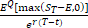(18)

- for Put: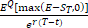(19)

3.2. Measuring the Entropy of Information of the Price of the UnderlyingAsset

Entropy of information measures the uncertainty associated with a random variable. The higher the value, the greater the uncertainty. It also measures the amount of information contained in the message and is expressed in bits.

Let X be a discreet random variable defined as such: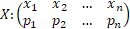, where. Then, the entropy of information of Shannon type is: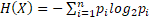(20)

If X is a continuous random variable, having the distribution density, then:(21)

### 4. Evaluation of Options with Compa SA Shares Underlying Asset using the Monte Carlo Simulation and the Entropy of Information

Next, we will empirically approach the valuation of options with non-dividend share underlying asset and measure the entropy of information for the price of the underlying asset, using the methodology described above.

We consider the underlying asset to be the shares of Compa SA listed on the Bucharest Stock Exchange. For the calculation of the volatility of the underlying asset we use data on the closing price of the share for July 19, 2017 - August 10, 2018 and the risk-free interest rate is 3.31% (BNR, 2018a).

Simulation of the price of Compa by the Monte Carlo method is performed starting with August 10, 2018, using equation (6). We created 252 daily scenarios for 252 days. The values of the Call and Put options for a Compa SA share - underlying asset are determined at 3 months, 6 months, 9 months and 12 months. See in this respect Tables 1, 2, 3, 4.

The entropy of information for the price of the underlying asset is measured annually over three years of trading. The values obtained are shown in Table 5.

Table 1. Call / Put value with 3-month maturity

 Time s1 s2 ……… s251 s252 CMP price - 10.08.2018 0.93 0.0000 0.9300 0.9300 ……… 0.9300 0.9300 Drift -0.2848 0.0040 0.9310 0.9182 ……… 0.9238 0.9370 Volatility 0.2903 0.0079 0.9254 0.8937 ……… 0.9189 0.9377 Time step (Δt) 0.0040 0.0119 0.9530 0.8917 ……… 0.9347 0.9100 Risk-free interest rate 0.0331 0.0159 0.9206 0.8867 ……… 0.9261 0.9280 Strike price (at the money) 0.93 0.0198 0.8983 0.9146 ……… 0.9360 0.9253 0.0238 0.9049 0.9375 ……… 0.9283 0.9532 0.0278 0.9026 0.9783 ……… 0.9405 0.9575 Value of the option: C 0.0708 0.0317 0.8897 0.9698 ……… 0.9684 0.9769 Value of the option: P 0.0447 0.0357 0.8780 0.9835 ……… 0.9508 1.0095 0.0397 0.8754 0.8754 ……… 0.9516 0.9928 0.0437 0.8735 0.9773 ……… 0.9477 1.0072 0.0476 0.8919 0.9531 ……… 0.9525 0.9990 ….. ….. ….. ……… ….. ….. 0.9960 0.5544 1.0197 ……… 0.9599 0.9313 1 0.5680 1.0358 ……… 0.9506 0.9215 3 months 0.25 0.8804 1.246157 ……… 0.898846 0.783104 CALL PAYOFF 0.0000 0.3162 0.0000 0.0000 PUT PAYOFF 0.0496 0.0000 0.0312 0.1469 CALL Average Payoff 0.0714 PUT Average Payoff 0.0451 CALL Value of the option: C 0.0708 PUT Value of the option: P 0.0447

Source: own calculations

Table 2. Call / Put value with 6-month maturity

 Time s1 s2 ……… s251 s252 CMP price - 10.08.2018 0.93 0.0000 0.9300 0.9300 ……… 0.9300 0.9300 Drift -0.2848 0.0040 0.9310 0.9182 ……… 0.9238 0.9370 Volatility 0.2903 0.0079 0.9254 0.8937 ……… 0.9189 0.9377 Time step (Δt) 0.0040 0.0119 0.9530 0.8917 ……… 0.9347 0.9100 Risk-free interest rate 0.0331 0.0159 0.9206 0.8867 ……… 0.9261 0.9280 Strike price (at the money) 0.93 0.0198 0.8983 0.9146 ……… 0.9360 0.9253 0.0238 0.9049 0.9375 ……… 0.9283 0.9532 0.0278 0.9026 0.9783 ……… 0.9405 0.9575 Value of the option: C 0.0934 0.0317 0.8897 0.9698 ……… 0.9684 0.9769 Value of the option: P 0.0633 0.0357 0.8780 0.9835 ……… 0.9508 1.0095 0.0397 0.8754 0.8754 ……… 0.9516 0.9928 0.0437 0.8735 0.9773 ……… 0.9477 1.0072 0.0476 0.8919 0.9531 ……… 0.9525 0.9990 ….. ….. ….. ……… ….. ….. 0.9960 0.5544 1.0197 ……… 0.9599 0.9313 1 0.5680 1.0358 ……… 0.9506 0.9215 6 months 0.5 0.8376 1.262709 ……… 0.929374 0.93227 CALL PAYOFF 0.0000 0.3327 0.0000 0.0023 PUT PAYOFF 0.0924 0.0000 0.0006 0.0000 CALL Average Payoff 0.0949 PUT Average Payoff 0.0643 CALL Value of the option: C 0.0934 PUT Value of the option: P 0.0633

Source: own calculations

Table 3. Call / Put value with 9-month maturity

 Time s1 s2 ……… s251 s252 CMP price - 10.08.2018 0.93 0.0000 0.9300 0.9300 ……… 0.9300 0.9300 Drift -0.2848 0.0040 0.9310 0.9182 ……… 0.9238 0.9370 Volatility 0.2903 0.0079 0.9254 0.8937 ……… 0.9189 0.9377 Time step (Δt) 0.0040 0.0119 0.9530 0.8917 ……… 0.9347 0.9100 Risk-free interest rate 0.0331 0.0159 0.9206 0.8867 ……… 0.9261 0.9280 Strike price (at the money) 0.93 0.0198 0.8983 0.9146 ……… 0.9360 0.9253 0.0238 0.9049 0.9375 ……… 0.9283 0.9532 0.0278 0.9026 0.9783 ……… 0.9405 0.9575 Value of the option: C 0.1096 0.0317 0.8897 0.9698 ……… 0.9684 0.9769 Value of the option: P 0.0766 0.0357 0.8780 0.9835 ……… 0.9508 1.0095 0.0397 0.8754 0.8754 ……… 0.9516 0.9928 0.0437 0.8735 0.9773 ……… 0.9477 1.0072 0.0476 0.8919 0.9531 ……… 0.9525 0.9990 ….. ….. ….. ……… ….. ….. 0.9960 0.5544 1.0197 ……… 0.9599 0.9313 1 0.5680 1.0358 ……… 0.9506 0.9215 9 months 0.75 0.5364 1.273412 ……… 1.108649 0.857565 CALL PAYOFF 0.0000 0.3434 0.1786 0.0000 PUT PAYOFF 0.3936 0.0000 0.0000 0.0724 CALL Average Payoff 0.1123 PUT Average Payoff 0.0785 CALL Value of the option: C 0.1096 PUT Value of the option: P 0.0766

Source: own calculation

Table 4. Call / Put value with 12-month maturity

 Time s1 s2 ……… s251 s252 CMP price - 10.08.2018 0.93 0.0000 0.9300 0.9300 ……… 0.9300 0.9300 Drift -0.2848 0.0040 0.9310 0.9182 ……… 0.9238 0.9370 Volatility 0.2903 0.0079 0.9254 0.8937 ……… 0.9189 0.9377 Time step (Δt) 0.0040 0.0119 0.9530 0.8917 ……… 0.9347 0.9100 Risk-free interest rate 0.0331 0.0159 0.9206 0.8867 ……… 0.9261 0.9280 Strike price (at the money) 0.93 0.0198 0.8983 0.9146 ……… 0.9360 0.9253 0.0238 0.9049 0.9375 ……… 0.9283 0.9532 0.0278 0.9026 0.9783 ……… 0.9405 0.9575 Value of the option: C 0.1403 0.0317 0.8897 0.9698 ……… 0.9684 0.9769 Value of the option: P 0.0822 0.0357 0.8780 0.9835 ……… 0.9508 1.0095 0.0397 0.8754 0.8754 ……… 0.9516 0.9928 0.0437 0.8735 0.9773 ……… 0.9477 1.0072 0.0476 0.8919 0.9531 ……… 0.9525 0.9990 ….. ….. ….. ……… ….. ….. 0.9960 0.5544 1.0197 ……… 0.9599 0.9313 1 0.5680 1.0358 ……… 0.9506 0.9215 12 months 1 0.5680 1.035832 ……… 0.950598 0.921524 CALL PAYOFF 0.0000 0.1058 0.0206 0.0000 PUT PAYOFF 0.3620 0.0000 0.0000 0.0085 CALL Average Payoff 0.1450 PUT Average Payoff 0.0850 CALL Value of the option: C 0.1403 PUT Value of the option: P 0.0822

Source: own calculations

Table 5. Shannon entropy

 No. Period Shannon entropy 1. 24.04.2015 – 11.07.2016 5.666305 2. 12.07.2016 – 18.07.2017 6.067939 3. 19.07.2017 – 10.08. 2018 6.069053

Source: own calculations

### 5. Conclusions

Following calculations, we can state the following:

a) The value of the Call option for a quantity of an underlying asset unit of the nature of Compa SA share is approximately:

- with 3-month maturity: 0.0708 monetary units (7.61% of the underlying asset on August 10, 2018);

- with 6-month maturity: 0.0934 monetary units (10.04% of the underlying asset on August 10, 2018);

- with 9-month maturity: 0.1096 monetary units (11.78% of the underlying asset on August 10, 2018);

- with 12-month maturity: 0.1403 monetary units (15.08% of the underlying asset on August 10, 2018).

b) The value of the Put option for a quantity of an underlying asset unit of the nature of Compa SA share is approximately:

- with 3-month maturity: 0.0447 monetary units (4.80% of the underlying asset on August 10, 2018);

- with 6-month maturity: 0.0633 monetary units (6.80% of the underlying asset on August 10, 2018);

- with 9-month maturity: 0.0766 monetary units (8.24% of the underlying asset on August 10, 2018);

- with 12-month maturity: 0.0822 monetary units (8.84% of the underlying asset on August 10, 2018).

c) The annual entropy of information for the underlying asset price is: 5,666305 for the period 24.04.2015 - 11.07.2016; 6,067939 for the period 12.07.2016 - 18.07.2017; 6,069053 for the period 19.07.2017 - 10.08.2018. An increase in uncertainty is observed dynamically.

###### References
1. Bentes, S. and Menezes, R., 2012. Entropy: A new measure of stock market volatility? Journal of Physics: Conference Series, 394(1): 012033, pp.1-6.
2. Bingqian, L., 2011. Monte Carlo Simulation and Option Pricing. Undergraduate Mathematics Department, Pennsylvania State University, USA. [online] Available at: http://www.personal.psu.edu/alm24/undergrad/bingqianMonteCarlo.pdf  [Accessed on 11 September 2018].
3. Black, F. and Scholes, M., 1973. The Pricing of Options and Corporate Liabilities. The Journal of Political Economy, Vol. 81, No. 3, pp. 637-654.
4. BNR, 2018a. Emisiune pe piata primara [online] Available at: http://www.bnr.ro/Emisiuni-pe-piata-primara-5651.aspx [Accessed on 11 September 2018].
5. BNR, 2018b. The National Bank of Romania [online]. Available at: www.bnr.ro [Accessed on 11 September 2018].
6. Bolia, N. and Juneja S., 2005. Monte Carlo Methods for Pricing Financial Options. Sādhanā, Vol. 30, Parts 2 & 3, pp. 347-385.
7. Boyle, P., 1977. Options: A Monte Carlo Approach. Journal of Financial Economics, Vol. 4, No. 3, pp. 323-338.
8. Brătian, V., 2017. Options Evaluation Using Monte Carlo Simulation. Revista Economică, Vol. 69, Nr. 4, pp. 30-42.
9. Brătian. V. (coordinator), Bucur, A. and Opreana, C., 2016. Finanțe cantitative Evaluarea valorilor mobiliare și gestiunea portofoliului. Editura ULBS, Sibiu.
10. Broadie, M. and Glasserman, P., 1996. Estimating Security Price Derivatives Using Simulation. Management Science, Vol. 42, No. 2, pp. 269-285.
11. BVB.ro, 2018a. Financial Instruments – Compa SA [online] Available at: http://www.bvb.ro/FinancialInstruments/Details/FinancialInstrumentsDetails.aspx?s=CMP [Accessed on 11 September 2018].
12. BVB.ro, 2018b. Bucharest Stock Exchange [online] Available at: www.bvb.ro [Accessed on 11 September 2018].
13. Cabrales, A., Gossner, O. and Serrano, R., 2011. Entropy and the value of information for investors. PSE Working Papers no 2011-40. [online] Available at: https://halshs.archives-ouvertes.fr/halshs-00648884/document [Accessed on 11 September 2018].
14. Charnes, J., 2000. Using Simulation for Option Pricing, in Proceeding of the Winter Simulation Conference, J. A. Joines, R. R. Barton, K. Kang and P. A. Fishwick, eds., Orlando, USA, pp151-157. 2000 Winter Simulation Conference, December 10–13, 2000, Wyndham Palace Resort & Spa, Orlando, FL, U.S.A., www.wintersim.org.
15. Giles, M., 2007. Monte Carlo evaluation of sensitivities in computational finance. Oxford University Computing Laboratory. [online] Available at: http://eprints.maths.ox.ac.uk/1090/1/NA-07-12.pdf [Accessed on 11 September 2018].
16. Jespersen, C., 2015. Monte Carlo Evaluation of Financial Options using a GPU. Master Thesis. Computer Science Department, Aarhus University, Denmark. [online]  Available online at: http://www.cs.au.dk/~gerth/advising/thesis/claus-jespersen.pdf [Accessed on 11 September 2018].
17. Longstaff, F. and Schwartz, E., 2001. Valuing American Options by Simulation: A Simple Least Squares Approach. Review of Financial Studies, Vol. 14, No. 1, pp. 113-147.
18. Maasoumi, E., Racine, J., 2002. Entropy and predictability of stock market returns. Journal of Econometrics, Vol.107, No. 1-2, pp. 291-312.
19. Nawrocki, D., Harding, W., 1986. State-Value Weighted Entropy as a Measure of Investment Risk. Applied Economics, Vol. 18, No. 4, pp. 411-419.
20. Negrea, B., 2006. Evaluarea activelor financiare – o introducere în teoria proceselor stocastice aplicate în finanțe. București: Editura Economică.
21. Negrea, B., Damian, V., 2015. Calcul stochastic aplicat în ingineria financiară. Editura Economică, București.
22. Nørretranders, T., 2009. Iluzia utilizatorului. București: Editura Economică.
23. Pele, D., Lazăr, E. and Dufour, A., 2017. Information Entropy and Measures of Market Risk. Entropy, Vol. 19, No. 5, pp. 1-19.
24. Shannon, C., 1948. A Mathematical Theory of Communication. The Bell System Technical Journal, Vol. 27, pp. 379-423, 623-656, July, October.
25. Sheraz, M., Dedu, S. and Preda, V., 2015. Entropy Measures for Assessing Volatile Markets. Procedia Economics and Finance, Vol. 22, pp. 655-662.
26. Verdú, S., 1998. Fifty Years of Shannon Theory. IEEE Transactions and Information Theory, Vol. 44, No. 6, pp. 2057-2078.
27. Wilmott, P., 2007. Introduces Quantitative Finance. Chichester, England: John Wiley & Sons.
28. Zhou, R., Cai, R., and Tong, G., 2013. Applications of Entropy in Finance: A Review. Entropy, Vol. 15, pp. 4909-4931.

© 2018 The Author. Published by Sprint Investify. ISSN 2359-7712. This article is licensed under a Creative Commons Attribution 4.0 International License.